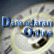##Debt Ratio Regression: January 2014

Variables used in the regression

1. Debt Ratio = Debt/ (Market Value of Equity + Debt): If you can get market value of debt, use it. Else, use book value of debt.
2. Expected growth in EPs= Expected annual growth rate in EPS over next five years
3. Regression Beta = Beta from a regression or a bottom up beta
4. EBITDA/ Enterprise Value = EBITDA/ (Market Value of Equity + Book value of Debt - Cash)

 Model R R Square Adjusted R Square Std. Error of the Estimate 1 .293a .086 .084 1365.48066% a. Predictors: (Constant), Regression Beta, EBITDA/Enterprise Value, Expected Growth in EPS (next 5 years)

 ANOVAa,b Model Sum of Squares df Mean Square F Sig. 1 Regression 323975514.43 3 107991838.14 57.919 .000c Residual 3462446037.2 1857 1864537.446 Total 3786421552.3 1860 a. Dependent Variable: Market Debt to Cap b. Weighted Least Squares Regression - Weighted by Market Cap c. Predictors: (Constant), Regression Beta, EBITDA/Enterprise Value, Expected Growth in EPS (next 5 years)

 Coefficientsa,b Model Unstandardized Coefficients Standardized Coefficients t Sig. B Std. Error Beta 1 (Constant) 0.1479 .01375 10.756 .000 EBITDA/Enterprise Value .374 .092 .101 4.075 .000 Expected Growth in EPS (next 5 years) -.396 .045 -.225 -8.769 .000 Regression Beta .0580 .0095 .139 6.060 .000 a. Dependent Variable: Market Debt to Cap b. Weighted Least Squares Regression - Weighted by Market Cap

• How do I use this regression?

Assume that you want to estimate the market debt ratio for a firm with the following characteristics:

EBITDA/ Enterprise Value = 8%

Expected growth rate in EPS = 10%

Regression Beta = 1.50

• Predicted Value

Expected Debt Ratio = 0.1479 + 0.374 *.08 + 0.058*1.50 - 0.396 *.10 = .2252 or 22.52%

If your predicted value is less than zero, your predicted debt ratio is zero.